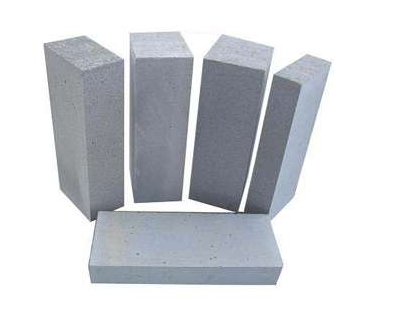# 混凝土配合比设计过程

## 混凝土配合比设计过程

2017-01-23 作者: xuzhiping 浏览: 4115 次

## 混凝土配合比怎么算

1、fcu,0=fcu+1.645σ=30+1.645×5.0=38.2Mpa。

2、w/c=aa×fce/(fcu,o+aa×ab×fce)=0.46×45.0/(38.2+0.46×0.07×45.0)=0.52

3、根据施工要求，混凝土设计坍落度为120mm~160mm，取单位用水量为215kg，掺加1.7%的缓凝高效减水剂，减水率δ=22%，则混凝土单位用水量：mW0= mW(1-δ)=215×(1-22%)=168kg。

4、单位水泥用量：mc=mwo/w/c=168/0.52=323kg

5、用粉煤灰取代16.5%的水泥，取代系数为λ=1.3, 则有 水泥用量：mc0= mc×(1-16.5%)=270 kg。粉煤灰用量：mf0= mc×16.5%×1.3=69.3kg。减水剂用量：mFDN0=( mc0+ mf0)×1.7%=5.77 kg。

6、假设混凝土单位容重mcp=2400kg/m，砂率βs=40%，则有：

mc0+ mf0+ ms0+ mg0+ mW0= mcp

mso/(mso+mgo) ×100%=βs

mc=mwo/w/c=168/0.49=343kg